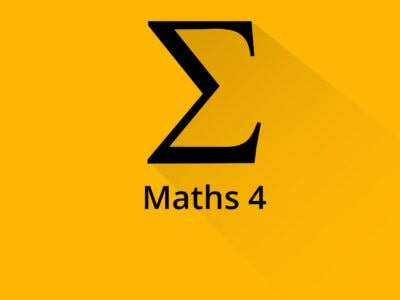Engineering Maths- 4 [All Branches] ( Videos + Handmade Notes ) AKTU(0 review)
₹1,500.00 ₹499.00Want to Clear your Engineering Math’s 4 in first attempt without any problem | Enroll this courses | Video’s for all branches in Hindi for Beginners

Branches Covered ( Comps , Mechanical , Civil , EXTC , Electrical , Electronics  )Handmade Notes : Notes are Brilliant , Easy Language , East to understand ( Student Feedback )Exam ke Pehle Notes ek baar Dekhlo revision aise hi jata hai

• Linear Algebra : Vector space

Engineering Mathematics-IV is semester 4 subject of final year of computer engineering in Mumbai University. Prerequisite for studying this subject are Engineering Mathematics-I, Engineering Mathematics-II, Engineering Mathematics-III, Binomial Distribution. Course Objectives of Engineering Mathematics-IV aims to learn Matrix algebra to understand engineering problems. Line and Contour integrals and expansion of a complex valued function in a power series.  Z-Transforms and Inverse Z-Transforms with its properties. The concepts of probability distributions and sampling theory for small samples.  Linear and Non-linear programming problems of optimization. Course Outcomes Engineering Mathematics-IV On successful completion, of course, learner/student will be able to Apply the concepts of eigenvalues and eigenvectors in engineering problems. Use the concepts of Complex Integration for evaluating integrals, computing residues & evaluate various contour integrals. Apply the concept of Z- transformation and inverse in engineering problems.  Use the concept of probability distribution and sampling theory to engineering problems. Apply the concept of Linear Programming Problems to optimization. Solve Non-Linear Programming Problems for optimization of engineering problems.

Module Linear Algebra (Theory of Matrices) consists of the following subtopics Characteristic Equation, Eigenvalues and Eigenvectors, and properties (without proof). Cayley-Hamilton Theorem (without proof), verification and reduction of higher degree polynomials. Similarity of matrices, diagonalizable and non-diagonalizable matrices. Self-learning Topics: Derogatory and non-derogatory matrices, Functions of Square Matrix, Linear Transformations, Quadratic forms.

Module Complex Integration consists of the following subtopics Line Integral, Cauchy‟s Integral theorem for simple connected and multiply connected regions (without proof), Cauchy‟s Integral formula (without proof). Taylor‟s and Laurent‟s series (without proof). Definition of Singularity, Zeroes, poles off(z), Residues, Cauchy‟s Residue Theorem (without proof). Self-learning Topics: Application of Residue Theorem to evaluate real integrations.

Module Z Transform consists of the following subtopics Definition and Region of Convergence, Transform of Standard Functions: {𝑘𝑛𝑎𝑘}, {𝑎|𝑘|}, { +𝑛𝐶. 𝑎𝑘}, {𝑐 𝑘sin(𝛼𝑘 + 𝛽)}, {𝑐 𝑘 sinh 𝛼𝑘}, {𝑐 𝑘 cosh 𝛼𝑘}.   Properties of Z Transform: Change of Scale, Shifting Property, Multiplication, and Division by k, Convolution theorem. Inverse Z transform: Partial Fraction Method, Convolution Method. Self-learning Topics: Initial value theorem, Final value theorem, Inverse of Z Transform by Binomial Expansion.

Module Probability Distribution and Sampling Theory consists of the following subtopics Probability Distribution: Poisson and Normal distribution. Sampling distribution, Test of Hypothesis, Level of Significance, Critical region, One-tailed, and two-tailed test, Degree of freedom. Students‟ t-distribution (Small sample). Test the significance of mean and Difference between the means of two samples. Chi-Square Test: Test of goodness of fit and independence of attributes, Contingency table. Self-learning Topics: Test significance for Large samples, Estimate parameters of a population, Yate‟s Correction.

Module Linear Programming Problems consists of the following subtopics Types of solutions, Standard and Canonical of LPP, Basic and Feasible solutions, slack variables, surplus variables, Simplex method.  Artificial variables, Big-M method (Method of penalty). Duality, Dual of LPP and Dual Simplex Method Self-learning Topics: Sensitivity Analysis, Two-Phase Simplex Method, Revised Simplex Method.

Module Nonlinear Programming Problems consists of the following subtopics NLPP with one equality constraint (two or three variables) using the method of Lagrange‟s multipliers. NLPP with two equality constraints. NLPP with inequality constraint: Kuhn-Tucker conditions. Self-learning Topics: Problems with two inequality constraints, Unconstrained optimization: One-dimensional search method (Golden Search method, Newton‟s method). Gradient Search method.

Suggested Reference books for subject Engineering Mathematics-IV from Mumbai university are as follows Erwin Kreyszig, “Advanced Engineering Mathematics”, John Wiley & Sons.  R. K. Jain and S. R. K. Iyengar, “Advanced Engineering Mathematics”, Narosa. Brown and Churchill, “Complex Variables and Applications”, McGraw-Hill Education. T. Veerarajan, “Probability, Statistics and Random Processes”, McGraw-Hill Education.  Hamdy A Taha, “Operations Research: An Introduction”, Pearson.  S.S. Rao, “Engineering Optimization: Theory and Practice”, Wiley-Blackwell.  Hira and Gupta, “Operations Research”, S. Chand Publication.

Course Features

• Lectures 35
• Quizzes 0
• Duration 50 hours
• Skill level All levels
• Language English
• Students 1
• Certificate No
• Assessments YesAverage Rating

0
0 rating

Detailed Rating

5 Star
0%
4 Star
0%
3 Star
0%
2 Star
0%
1 Star
0%